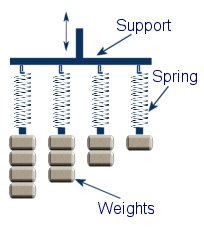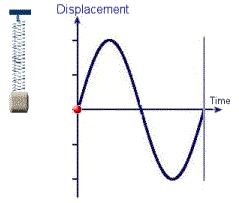WOOCS 1.2.7.1
01270 747 008 (UK)

Resonance and Simple Harmonic MotionThis experiments is a simple way to demonstrate the principles of resonance and simple harmonic motion.

This diagram show four identical springs attached to a support. Each spring has weight with a different mass attached to the end. If a weight is pulled and released, it will set it in motion bouncing up and down until all its energy is dissipated through friction. As each spring holds a different mass, they will each have a different fundamental or resonant frequency. The first spring with the most mass will bounce slowest. We say that this has the lowest resonant frequency out of the four springs. The frequency is simply the number of times the mass bounces up and down each second and is measured in Hertz (Hz).

If the support holding the springs is moved up and down at a set speed, we will see the weights begin to move up and down buy different amounts. The amount of displacement of each mass will be dependant upon the frequency that the support is oscillating at.We will see the maximum displacement when the frequency of the moving support is the same as the resonant frequency of one of the weights. For example; If the resonant frequency of the first mass and spring is 10Hz then it will move the most when the support is moved up and down at 10Hz. This is known as resonance. If the support is moving at 9Hz or 11Hz then there will be significantly less movement of the mass. This is because resonance usually occurs when the frequencies match almost exactly.

The exact same principles are used in radios and televisions in order to tune into a specific station. Instead of oscillating springs, it is the electrical currents that are oscillating. By using different components it is possible to make a circuit that has a resonant frequency that is the same as the radio wave that we wish to detect. At resonance the amplitude of the signal in the circuit will be much higher than any of the other signals which allow us to use just one channel at a time.

1.Perhaps your local DIY store will have some springs. You could use large bolts or bags of coins for the weights.

2.Where can I obtain the supplies to do this experiment?

3.No. The total kinetic energy in the system will only be equal to or less than the energy you put in.

4.I understand most of this, but the question is can we use the movement of the spring to create energy?